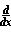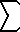(Math)

# An Introduction to Generalized Calculus

David Manura, questions, http://www.sisweb.com/math/tables.htm, August 1997.

The following was derived a couple of years ago therefore notation and methods are lax. I do not know how instrumental this is in broadening the realm of calculus or useful since many results relations revert to elementary calculus. I am still searching for an application for this theory. Write to me at the above address with comments.# Preface

Elementary calculus is only one many calculuses, characterized by a line, but there are other non-linear calculus's which have their own set of properties. To investigate these non-linear calculus's, we need to define what a calculus is.

Definition of a calculus: A calculus is a system composed of the four building blocks: the discrete limit, continuous limit, discrete iteration and continuous iteration.
 The Building Blocks of a Calculus Discrete Limit * Continuous Limit Discrete Iteration Continuous Iteration
* This paper will not investigate the discrete limit to much depth.

For elementary calculus, these building blocks are:

• Discrete Limit: <unknown>.
• Continuous Limit: Derivative• Discrete Iteration: Summation• Continuous Iteration: IntegralWe can chart the elementary calculus as:
 Elementary Calculus Continuous Discrete LimitIterationNow we will define what these indivual building blocks are so that we can create new ones, and from there create new calculuses. Only informal definitions are given.

Continuous Limit: This is a limit expressing change in a function over an infinitely small interval. It need not be linear change, as in the derivative, but may be any infinitesimal relationship.

Discrete Iteration: As its name implies, it is a repeated opperation performed a finite number of times in a finite interval. It need not be the sum of all the values of a function when given integer parameters, as in the summation, but may be any iterative opperation such as a product or continued fraction.

Continuous Iteration: This is the Discrete Iteration made continuous. There are set rules to convert a discrete iteration into its continuous counterpart. A continuous iteration can be expressed in terms of an infinite limit of the corresponding discrete iteration. This concept can be seen in the case of the integral, which is the summation of partitions under a function as the size of the chosen partitions approaches zero.

>>> The continuous iteration and the continuous limit are always inverse opperations of each other. In the case of linear (elementary) calculus, the integral and derivative have inversive properties as defined by the Fundamental Theorem of Calculus. <<<

The Discrete Limit: I have not yet worked out a theory on this building block. By intuition, I assume it exists. I define it to be the inverse opperation of the discrete iteration and express the finite change (not neccessarily linear change) of a function in a finite interval. This may be [ f(x+1)-f(x) ] / 1 for linear calculus, but I have not verified that hypothysis.

Note the relations between these buildng blocks:

• The continuous limit and continuous iteration are inverse functions of each other.
• The continuous iteration is the discrete iteration made continuous . We can express the continuous iteration as a limit of the corresponding discrete iteration.
Now since we have delineated what a calculus is, we can expolore our first non-linear calculus in the next section.